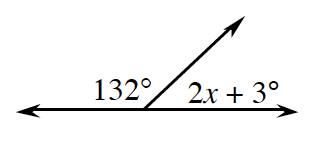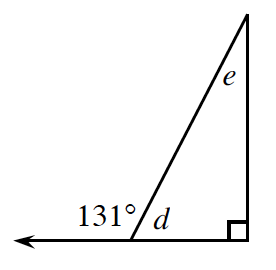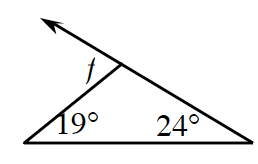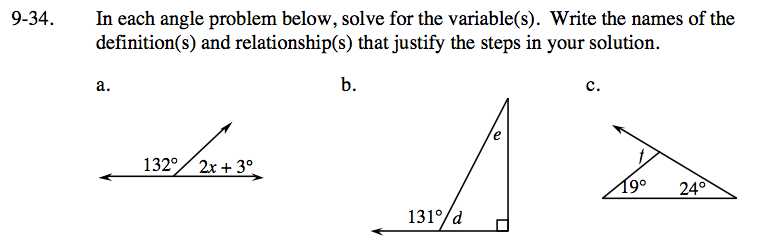Home > ACC7 > Chapter cc39 > Lesson cc39.1.3 > Problem9-34

9-34.
1. In each angle problem below, solve for the variable(s). Write the names of the definition(s) and relationship(s) that justify the steps in your solution. Homework Help ✎

2.  a.b.c.132 + (2x + 3) = 180

The angle measuring 131° is an exterior angle to the triangle.

d + 131 = 180

d = 49°

d + e + 90 = 180
or
131 = e + 90

e = 41°

An exterior angle is equal to the sum of the remote interior angles.